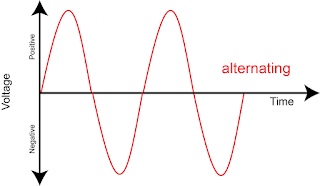# Principle of AC generator class 12th (Explained)

What comes into your mind, when you hear the term “Alternating Current“? Let me guess, a sort of electricity or something ?

Yes you are correct but in this article, we are not only going to look at alternating current but how alternating current is generated a.k.a AC generator and the working principle of the AC generator.

The principle of Alternating Current (A.C) generators is electromagnetic induction and Faraday’s law. Magnetic flux is induced in a coil due to which electric current starts to flow but there are some other things as well necessary for current to flow.

## What is alternating current ?

Alternating current is a current which reverses its direction periodically unlike direct current which doesn’t change its direction. The graph of alternating current is sinusoidal whereas of direct current the graph is straight.Graph of alternating current

Now, you at least have an idea of what alternating current is like. So before going ahead, you should know that alternating current is what we use in our homes and in our day to day life because transportation of electricity is a lot more due to alternating current.

P.s:- Alternating current is more dangerous than direct current.

## What is alternating current (A.C) generator ?

Alternating current generator or A.C generator as the name suggests is the device used to generate alternating current (A.C).

The main work of this device is to convert mechanical energy into electrical energy and this electrical energy is what we call as alternating current.

## What is the principle of the AC generator ? How it works ?

Alternating current generator basically depends upon the principle of electromagnetic induction. The mechanism of this device is such that it creates changing magnetic flux passing through a coil due to which current is induced in a coil.

The current which is used to power up our houses is alternating current.

You may be wondering about electromagnetic induction, but before getting straight into it you need to know about Faraday’s law for better understanding.

### What is Faraday’s law ?

Faraday proposed two laws known as Faraday’s law of electromagnetic induction. To understand Faraday’s law the term you need to understand is magnetic flux.

#### Magnetic flux:

Magnetic flux is the amount of magnetic field line passing through a coil. This magnetic flux is the reason due to which the AC generator is able to generate alternating current.

1. Whenever there is a change in the magnetic flux linked to a coil, an E.M.F is linked across a coil and if the circuit is closed, current starts flowing.

Basically, first law of Faraday says that change in magnetic flux linked to coil creates current flow in a coil if the circuit is closed.Faraday’s law

2. The magnitude of induced E.M.F across a coil is equal to the rate of change of magnetic flux linked to it.

P.s: negative sign ‘-‘ shows that E.M.F opposes the change in the flux.

For one turn of the coil, EMF induced will be:

P.s: B is the magnetic field intensity or magnetic flux and A is the area.

For ‘n’ numbers of turn, EMF induced will be:

If you put this in simpler words it just means that more EMF will be induced if the rate of change in magnetic flux is increased.

### What is electromagnetic induction ?

Electromagnetic induction is the phenomenon of the production of EMF across a conductor when there is a change in magnetic flux linked to it.Electromagnetic Induction

If the circuit is closed due to production of EMF, current will start flowing.

#### Electromagnetic induction role in AC generator:

In the working of alternating current (A.C) generator flux linked to a coil changes periodically due to the rotation of a coil by mechanical energy, due to this, rate of change of magnetic flux also changes periodically resulting in the production of alternating current.

## Conclusion:

So AC Generator depends on the rate of change in the amount of flux linking to it and this phenomenon is also known as Electromagnetic Induction.

There are two laws of electromagnetic induction given by Faraday known as Faraday’s law of electromagnetic induction.

### F.A.Q:

Q. What is magnetic flux ?

It’s the intensity of magnetic filed lines passing through a particular area.

Q. Will EMF always get induced if magnetic flux is there ?

No EMF will only be induced when there is a change in the amount of magnetic flux it doesn’t depend whether magnetic flux is increasing or decreasing. The key point is, there should be a change in magnetic flux.

Q. Why EMF gets induced when there is a change in magnetic flux ?

Nature wants to oppose the change by default. We all know that flowing current creates a magnetic field, so when there is a change in magnetic flux linked to a coil to oppose that change nature induces EMF which makes the current flow and opposing magnetic fields are created which opposes the change in a magnetic field. This is called Lenz law.

So to oppose the change in magnetic flux nature induces EMF across the coil.

## 2 thoughts on “Principle of AC generator class 12th (Explained)”

1.Sheetal Prajapati says:

Amazing notes🔥😌❤️

2.Abhay Kumar says: